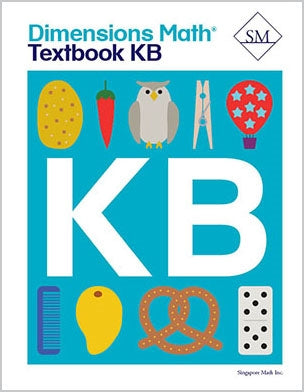# Dimensions Math Textbook KB

\$ 12.00

Dimensions Math® Textbook KB

Chapter 7: Numbers to 20

Lesson 1: Ten and Some More
Lesson 2: Count Ten and Some More
Lesson 3: Two Ways to Count
Lesson 4: Numbers 16 to 20
Lesson 5: Number Words 0 to 10
Lesson 6: Number Words 11 to 15
Lesson 7: Number Words 16 to 20
Lesson 8: Number Order
Lesson 9: 1 More Than or Less Than
Lesson 10: Practice — Part 1
Lesson 11: Practice — Part 2

Chapter 8: Number Bonds

Lesson 1: Putting Numbers Together — Part 1
Lesson 2: Putting Numbers Together — Part 2
Lesson 3: Parts Making a Whole
Lesson 4: Look for a Part
Lesson 5: Number Bonds for 2, 3, and 4
Lesson 6: Number Bonds for 5
Lesson 7: Number Bonds for 6
Lesson 8: Number Bonds for 7
Lesson 9: Number Bonds for 8
Lesson 10: Number Bonds for 9
Lesson 11: Number Bonds for 10
Lesson 12: Practice — Part 1
Lesson 13: Practice — Part 2
Lesson 14: Practice — Part 3

Lesson 1: Introduction to Addition — Part 1
Lesson 2: Introduction to Addition — Part 2
Lesson 3: Introduction to Addition — Part 3
Lesson 5: Count On — Part 1
Lesson 6: Count On — Part 2
Lesson 7: Add Up to 3 and 4
Lesson 8: Add Up to 5 and 6
Lesson 9: Add Up to 7 and 8
Lesson 10: Add Up to 9 and 10
Lesson 12: Practice

Chapter 10: Subtraction

Lesson 1: Take Away to Subtract — Part 1
Lesson 2: Take Away to Subtract — Part 2
Lesson 3: Take Away to Subtract — Part 3
Lesson 4: Take Apart to Subtract — Part 1
Lesson 5: Take Apart to Subtract — Part 2
Lesson 6: Count Back
Lesson 7: Subtract Within 5
Lesson 8: Subtract Within 10 — Part 1
Lesson 9: Subtract Within 10 — Part 2
Lesson 10: Practice

Lesson 2: Practice Addition and Subtraction
Lesson 3: Part-Whole Addition and Subtraction
Lesson 4: Add to or Take Away
Lesson 5: Put Together or Take Apart
Lesson 6: Practice

Chapter 12: Numbers to 100

Lesson 1: Count by Tens — Part 1
Lesson 2: Count by Tens — Part 2
Lesson 3: Numbers to 30
Lesson 4: Numbers to 40
Lesson 5: Numbers to 50
Lesson 6: Numbers to 80
Lesson 7: Numbers to 100 — Part 1
Lesson 8: Numbers to 100 — Part 2
Lesson 9: Count by Fives — Part 1
Lesson 10: Count by Fives — Part 2
Lesson 11: Practice

Chapter 13: Time

Lesson 1: Day and Night
Lesson 2: Learning About the Clock
Lesson 3: Telling Time to the Hour — Part 1
Lesson 4: Telling Time to the Hour — Part 2
Lesson 5: Practice

Chapter 14: Money

Lesson 1: Coins
Lesson 2: Pennies
Lesson 3: Nickels
Lesson 4: Dimes
Lesson 5: Quarters
Lesson 6: Practice

Dimensions Math® PreK-5 series features the progression, rigor, and pacing that define Singapore math. Throughout the series, five characters offer students suggestions on how to think about problems. They remind students of strategies they’ve learned and point out important information that encourages them to come up with their own solutions.

Textbook lessons begin with a task that allows students to apply their previous knowledge and learn through discussion. Once students have mastered a concept with the use of concrete and pictorial aids, they are ready to take on more abstract mathematical problem sets. They reach fluency by collecting various strategies along the way and applying them to new problems. Word problems give students a sense of math in real-world contexts.

Workbooks offer independent practice that follows a careful progression of exercise variation. Each textbook lesson includes a corresponding workbook exercise that starts with pictorial representation and progresses to more challenging abstract problems. Workbooks for PreK-2 are perforated.

Teacher’s Guides include lesson plans, mathematical background, games, helpful suggestions, and comprehensive resources for daily lessons. Lessons are laid out clearly and activities are designed for the whole class, small groups, and extension.

Textbooks and Workbooks do not include answer keys. Answers are in Teacher's Guides.
Textbook:
ISBN 9781947226036
pp 200